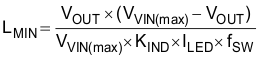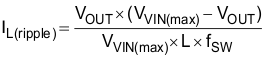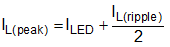SLUSCO8B November   2016  – June 2018

PRODUCTION DATA.

1. Features
2. Applications
3. Description
1.     Device Images
4. Revision History
5. Description (continued)
6. Pin Configuration and Functions
7. Specifications
8. Detailed Description
1. 8.1 Overview
2. 8.2 Functional Block Diagram
3. 8.3 Feature Description
4. 8.4 Device Functional Modes
9. Application and Implementation
1. 9.1 Application Information
2. 9.2 Typical Application
1. 9.2.1 TPS5420x 12-V Input, 1.5-A, 3-Piece IR LED Driver With Analog Dimming
2. 9.2.2 TPS5420x 24-V Input, 1-A, 4-Piece WLED Driver With PWM Dimming
10. 10Power Supply Recommendations
11. 11Layout
12. 12Device and Documentation Support
13. 13Mechanical, Packaging, and Orderable Information

• DDC|6

#### 9.2.1.2.1 Inductor Selection

Use Equation 3 to calculate the minimum value of the output inductor (LMIN).

Equation 3.where

• KIND is a coefficient that represents the amount of inductor ripple current relative to the maximum LED current.
• ILED is the maximum LED current.
• VOUT is the sum of the voltage across LED load and the voltage across the sense resistor.

In general, the suggested value of KIND is between 0.2 and 0.4. For an application that can tolerate higher LED current ripple or use larger output capacitors, one can choose 0.4 for KIND. Otherwise, a smaller KIND like 0.2 can be chosen to get low-enough LED current ripple.

With the chosen inductor value the user can calculate the actual inductor current ripple using Equation 4.

Equation 4.The inductor rms-current and saturation-current ratings must be greater than the rms current and saturation current seen in the application. This ensures that the inductor does not overheat or saturate. During power up, transient conditions, or fault conditions, the inductor current can exceed its normal operating current. For this reason, the most conservative approach is to specify an inductor with a saturation current rating equal to or greater than the converter current limit. This is not always possible due to application size limitations. The peak-inductor-current and rms-current equations are shown in Equation 5 and Equation 6.

Equation 5.Equation 6.In this design, choose KIND = 0.3. According to the LED manufacturer’s data sheet, the IR LED has 1.75-V forward voltage at 1.5-A current, so VOUT = 1.75 V × 3 + 0.2 V = 5.45 V and the calculated inductance is 11.9 µH. A 10-µH inductor (part number is 744066100 from Wurth) is chosen. With this inductor, the ripple, peak, and rms currents of the inductor are 0.53 A, 1.77 A, and 1.51 A, respectively. The chosen inductor has ample margin.